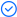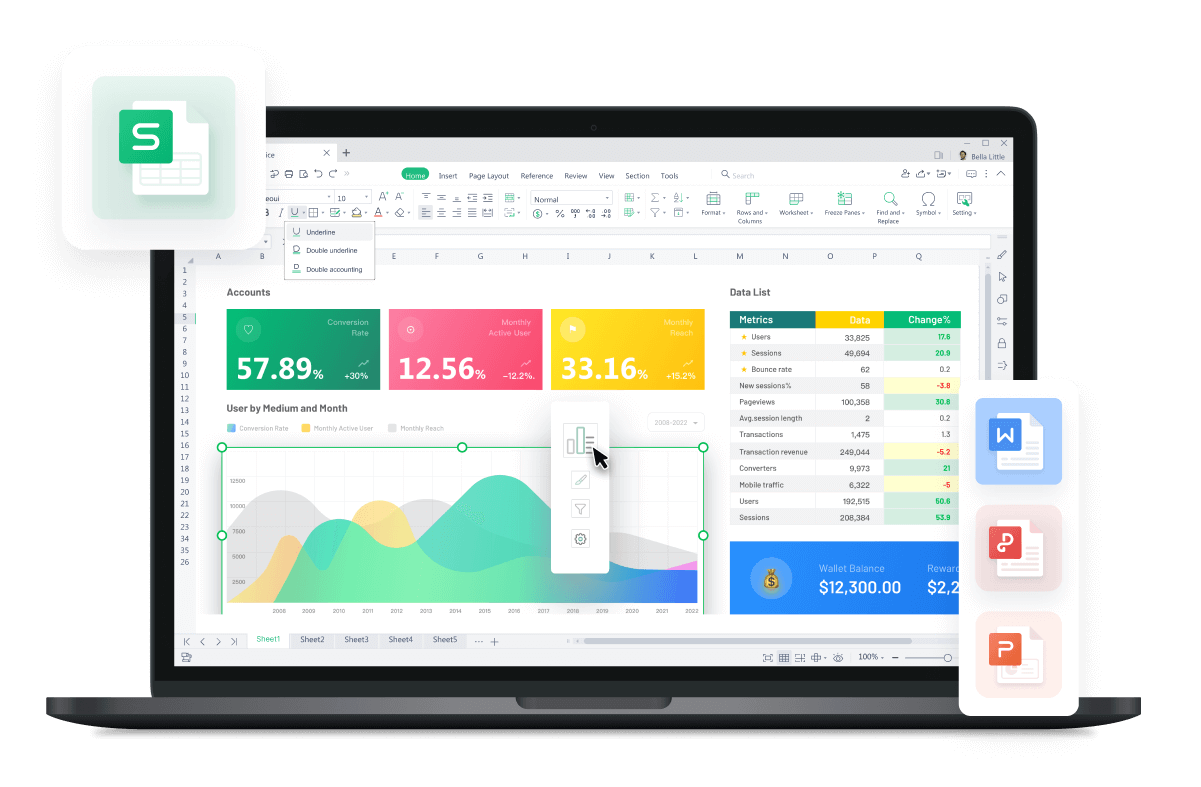WPS Office

Free All-in-One Office Suite with PDF EditorRead, edit, and convert PDFs with the powerful PDF toolkit.Microsoft-like interface, easy to use.

Windows • MacOS • Linux • iOS • Android# How to Compare the Values of Two Cells in Excel

August 9, 2022
3.5K Views

While working in Excel/Spreadsheet WPS, there are times when we need to compare the values of different cells. This becomes very difficult when the amount of data is huge in Excel/Spreadsheet WPS and we have a short time to complete this task. Comparing values of cells in Excel/Spreadsheet WPS(2016/2019/Mac/Online) helps in understanding the data and reduces a lot of repetition in work. Usually, a lot of people do not know How To Compare Values of Two Cells in Excel/Spreadsheet (2016/2019/Mac/Online). There are different ways to achieve this task.  After following the below-mentioned steps, anyone can easily compare values of two cells in Excel/Spreadsheet WPS(2016/2019/Mac/Online).

Excel/Spreadsheet is an easy-to-use tool that helps its users to save hours of work and they can complete tasks within a few minutes. The following steps will help you understand How to compare values of two cells in Excel/Spreadsheet WPS. After reading all the steps, you will be able to compare values of two cells in excel/spreadsheet WPS (2016/2019/Mac/Online) easily.

Different Methods to Compare Values of Two Cells in Excel/Spreadsheet WPS

How to Compare Two Cells in Excel/Spreadsheet WPS Using Equal (=) Sign

1.Open the desired worksheet in Spreadsheets (WPS Office) in which the two cells are required to be compared.

2.Use the equal sign = to compare the values of two cells as shown below.

3.If the values of the two cells are same then the result will be TRUE.

4.If the values of the two cells are not same then the result will be FALSE.

Note: In this method, the excel sheet does not differentiate between upper case and lower case letters.

How to Compare Two Cells in Excel/Spreadsheet WPS Using EXACT Command

1.In case the upper/lower case of letters is required to be considered then the EXACT command is used, as shown below.

2.If the letters are of the same case then the result will show TRUE otherwise it will show FALSE.

Note: This method does not check the style of text in the cells i.e. Bold, Italic, etc.

How to Compare Two Cells in Excel/Spreadsheet WPS using LEFT/RIGHT Command

1.If only a portion of the cells is required to be compared then it can be done by using the LEFT & RIGHT command.

For Example, if the 4 characters from LEFT/RIGHT of the cells are to be compared then it shall be done as shown below.

2.In case the upper/lower case of letters is also required to be checked then EXACT command is used with LEFT/RIGHT command, as shown below.

3.For the above example, if the first 4 characters in the two cells are same and have same letter case (upper/lower) then the result will be TRUE otherwise it will be FALSE.

This article has covered the methods on How to compare the values of two cells in excel/spreadsheet WPS (2016/2019/Mac/Online) using equal sign, how to compare the values of two cells in excel/spreadsheet WPS (2016/2019/Mac/Online) using the EXACT command and how to compare the values of two cells in excel/spreadsheet WPS (2016/2019/Mac/Online) using the LEFT/RIGHT command. You can use these simple steps as explained above and easily compare the values of two cells in your WPS Excel/Spreadsheet (2016/2019/Mac/Online) whenever required.